Next: Bibliography

Research Summary and Plan
Lawren Smithline

My research on algorithms applies to two general areas, mathematical biology and number theory. My switch to biological problems is recent. The broader impact of extracting information from biological sequences is great, despite the difficulty of precise problem definition. My graduate thesis develops a specialized number theory algorithm which converts a question about modular forms into a combinatorial problem. The basic problem of finding the prime divisors of an integer is a continuing interest of mine. I continue to consult for Institute for Defense Analyses (IDA), due to the application of number theory to cryptography.

I plan to continue developing biological sequence comparison algorithms. Goals include gaining insight into the process of evolution and understanding the semantics of the genetic code.

In the immediate future, I will refine the methods I describe below. Probabilitistic pairwise visualization (PPV) allows sequences to be compared using a data array which translates into an image showing regions of higher and lower correlation. The numerical data can then be processed by digital image techniques. It is a challenging programming problem to generalize to multiple sequences. Traceback and realignment of gaps (TAR) is a separate algorithm designed as part of an improvement to the CLUSTAL progressive multiple sequence alignment.

These methods have been tested on data from mouse, rat, and yeast. They can each benefit from development of stronger underlying models of evolution. I would like to use them to go beyond the information developed using other strategies, examining both larger and more complex data sets.

In my Ph. D. thesis [Sm], I develop a technique to analyze the slopes of the Atkin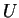operator, which acts as a compact operator on certain spaces of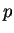-adic modular forms. In a later paper, I define the set of compact operators with rational generation (CORG). I show thatis a CORG and that the set of CORGs is an algebra. Based on numerical experiments, I conjecture structure in the slopes of these operators, which are proved, by me and others, in particular cases. The general case remains an open problem.

Mathematical Biology

Biological sequences are strings from an alphabet of four nucleotides or twenty amino acids. For simplicity, I will use nucleotide sequences as an example. DNA, the molecule of heredity in every cellular life form on Earth, is a polymer composed of two complementary chains of nucleotides. It encodes the instructions for every life process. The duplication process between generations is imperfect. The mutations may result in variations of individuals within species and, eventually, speciation.

My start on this project came from the suggestion of Jade Vinson at the Whitehead Institute at MIT in the summer of 2002, instigated by our common work on algorithms at IDA. I have been fortunate to have his advice, as well as that of Nick Patterson at Whitehead and Rick Durrett at Cornell.

In [Sm5], I describe a new algorithm, PPV, for visualizing an alignment of biological sequences according to a probabilistic model of evolution. The resulting data array is readily interpreted by the human eye and amenable to digital image techniques as well as statistical methods. The demonstration implementation uses an underlying evolutionary model derived from one proposed by Thorne, Kishino, and Felsenstein in [TKF] and improved by Hein and others in [H+].

PPV as implemented applies to two sequences and requires time and memory proportional to the product of the sequence lengths. There is a natural extension of PPV to multiple sequences using a result of [L+]. I describe a basic method to reduce the time and memory demands.

Along with the description of the algorithm, I present examples using mRNA sequences from mouse and rat. Figure 1, excerpted from [Sm5] and appearing after the list of references, is part of the analysis of two zinc finger proteins from chromosome 7 of Mus musculus. The many diagonal bands reflect the repeated 28 amino acid zinc finger functional units, with some more similar to each other. Evaluation of this kind of multiple similarity is a shortcoming of standard Smith-Waterman type dynamic programming methods, including CLUSTAL. For this example, BLAST finds only some of the multiple similarities, and does not assemble them into the larger picture.

The insight of [H+], discussed further in [L+], is the description of a Markov process for evolution using one main state and multiple transitions. This simplifies computing the total likelihood of two sequences aligning by any path, rather than solely the Viterbi maximum score path.

I extend their work by computing total likelihood from both the head and tail ends of sequences and combining the answers. The result is an array that compares sequences point by point by assigning a value to every ordered pair of loci, one in each sequence. The value is meaningful as a probability, comparable across an array, and together with a normalization I describe, comparable between arrays generated for different pairs of sequences. I explore a way to express degree of preference for local similarity by suggesting a model for jump'' events, where the sequence continuity possibly breaks and is not modeled well by insertion, deletion, and substitution.

I determine the equilbrium value,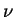for the array computed with random sequences. By assigning the valueto certain parts of the array compued for actual data, I can model the assumption that certain sequence comparisons verify the null hypothesis of no evolutionary relation. With this kind of masking, it will be possible to extend the algorithm in [Sm5] to longer sequences and multiple sequences.

For biological sequences with expected one to one correspondence, dynamic programming with Viterbi path computation is a good strategy to find the best alignment. Multiple sequence alignment (MSA) is used to detect candidates for functional regions of DNA. Within a species, genes that are part of the same metabolic pathway may have control regions which respond to the same regulating factors. Across species, parsimony predicts that homologous genes conserve essential functional groups.

CLUSTAL is a popular program for progressive MSA using Viterbi score paths. Given a set of sequences, CLUSTAL constructs a (binary) guide tree using a distance matrix from pairwise alignment and Saitou-Nei neighbor joining [SN]. Each leaf of the tree corresponds to a data sequence. For each interior node, when both of its children are labeled either by a single sequence or a MSA, the two objects are aligned pairwise by Smith-Waterman dynamic programming [SW]. The entries in the score matrix are computed by scoring the profiles of the two inputs. The parent node gets for a label the larger MSA, with a row corresponding to each row of each child. When the root of the tree gets a label, that grand MSA contains a row for every input sequence.

One well known limitation of CLUSTAL is its inability to backtrack on the tree; all partial MSAs are frozen. This can lead to suboptimal alignments due to gap position ambiguity. Here is an example where the improvement is obvious. Consider excerpts of three sequences

 X ATCCGAGATCGCGATCGA Y ATCCGA---GATCGA Z ATCCGTGATCGCTTCCGA

where, based on the whole sequence, X and Y are more closely related than either is to Z. In the excerpt shown, the score of the XY alignment is unchanged if the gap and the GAT following are swapped. Smith-Waterman alignment chooses one way. In light of sequence Z, we see that the alternative GAT followed by the gap is better. A transposable element often inserts following a string similar to its tail, leading to this kind of ambiguity. CLUSTAL has equal difficulty with the inverse situation, where the fragment on the edge of a gap is dissimilar from the opposite sequence on each side of the gap.

I propose a modification of the record kept by the dynamic programming segment, which allows traceback through the guide tree and realignment of gaps (TAR). At each stage of the progressive MSA, TAR attaches to every gap a range in which to float. At a later stage involving that alignment, that gap is floated in its range to optimize the score of the new MSA. TAR requires parameters to determine the range of the float and the trade-off between the score of the smaller alignment versus the score of the larger alignment.

The motivation for TAR comes from scanning CLUSTAL alignments for the biggest, easiest improvement. The idea to use a local method, relative to the guide tree, comes from the view of sequence alignments as reconstructions of evolutionary history. TAR overcomes the challenge presented by transposable elements. to multiple alignments.

A basic implementation of TAR, tested on a handful of cases shows a modest quantitative improvement over CLUSTAL, as measured by the recalculated CLUSTAL score, and a qualitative improvement in that the new alignments do not suggest obvious improvements. TAR applies to the Tcoffee progressive MSA algorithm [NHH]. Tcoffee also freezes intermediate MSAs. A natural future direction for TAR is complete implementation of TAR as an extension of CLUSTAL, Tcoffee, or any other progressive MSA method.

Number Theory

My interest in computation number theory began in about 1987, when I corresponded with Larry Carter, then at IBM, about enumerating prime numbers faster than the Sieve of Eratosthenes. Later, as an undergraduate, I wrote a senior thesis, Modern integer factorization under Noam Elkies, describing subexponential integer factorization algorithms, with emphasis on the number field sieve. IDA kept a copy of this paper for their library.

I continued with number theory in Berkeley. I wrote my dissertation on-adic modular forms under the direction of Robert Coleman.

A nontechnical sketch of the construction of modular forms begins with elliptic curves, which are cubic curves. When the complex numbers is the ground field of definition, an elliptic curve may be transformed by projective 2-space isomorphism to have the equation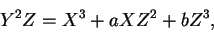for constants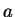and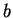.

An elliptic curve is an example of a algebraic group. The group law is that three collinear points on an elliptic curve sum to zero. In [Sm], [Sm2], [Sm3] and [Sm4], the ground field is-adic, and the elliptic curves may be marked with extra data, the level structure. For example, level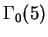structure adds to an elliptic curve a distinguished subgroup of order 5.

A modular curve is a parameter space for elliptic curves with a particular level structure. Differentials on this modular curve are modular forms. The weight of a modular form is twice the degree of the differential. Maps between modular curves lift to maps between modular forms which preserve weight. For example, there is a natural map from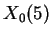, the space of elliptic curves with marked subgroup of order 5, to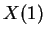, the space of elliptic curves with no extra structure, by ignoring the level structure. A modular form onpulls back to a modular form on.

Among the endomorphisms of modular forms is the Hecke algebra, which arises from traces of certain maps of modular curves. The modular forms derived from elliptic curves over a-adic ground field have a generalization, the overconvergent modular forms, such that the ideal generated by the Atkinoperator in the Hecke algebra is a set of compact operators on a-adic Banach space.

A compact operator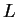has a spectrum which is the set of reciprocals of roots of the characteristic power series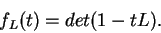Koike's formula computes the coefficients of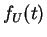. Their-adic valuations determine the valuations of the eigenvalues of. The Newton polygon ofis the lower convex hull of a plot of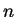versus the valuation of the coefficient of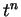in. The slopes of the Newton polygon are the valuations of eigenvalues.

I compute-adic approximations to theoperator when the related modular curve has genus zero. In this case, the ring of weight zero modular forms is a restricted power series ring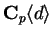, meaning there is a single weight zero modular form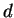such that every weight zero modular form may be realized as a power series inwith coefficients in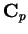such that power series converges on the closed disk of radius 1.

I determine a recursion forapplied to powers ofand realizeas an explicit infinite order matrix. (The same procedure is possible for arbitrary genus modular curves; the choice of module basis more difficult.)

Computational linear algebra applies to the matrix for. The operatoris compact, so truncations of the matrix converge in the-adic topology to. I prove that every instance ofhas a Newton polygon which is bounded below by a parabola. For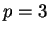and weight 0, I find a bounding parabola which coincides with the Newton polygon infinitely often and also the points of coincidence. The explicit results support the view of Gouvêa and Mazur that the slopes ofin different weights are related; however, there is a recent counterexample to their specific conjecture in [GM].

The good-adic approximations allow computation of many slopes of theoperator. The calculations are determinants of integer matrices, often with many zero entries. These calculations are faster than Koike's formula. On a Intel 486 machine using the Pari algebra system, we computed over a hundred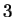-adic slopes using the matrix and its recursion, compared with less than forty for Koike's formula. The calculations with integer matrices would also be much easier to program without an algebra package than would the quotients of-adic algebraic numbers required by Koike's formula.

Buzzard, Calegari, and Kilford have done more extensive calculations for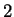-adic modular forms. See [Ki] for reference to all three. Using the recursive generation for the matrix and some ad hoc combinatorics, they have determined, with proof, some infinite sequences of slopes of some-adic modular forms. The short recursion makes realization of a closed form solution for the matrix coefficients easier than for larger primes.

The set of CORGs is an algebra. The Newton polygon of a CORG is bounded below by a parabola. Numerical experiments suggest that the Newton polygons are also bounded above by a parabola, with the same quadratic term; the difference between the bounding parabola and the Newton polygon grows linearly; and the slopes grow linearly, with a deviation that grows logarithmically.Next: Bibliography
Lawren Smithline 2003-11-29